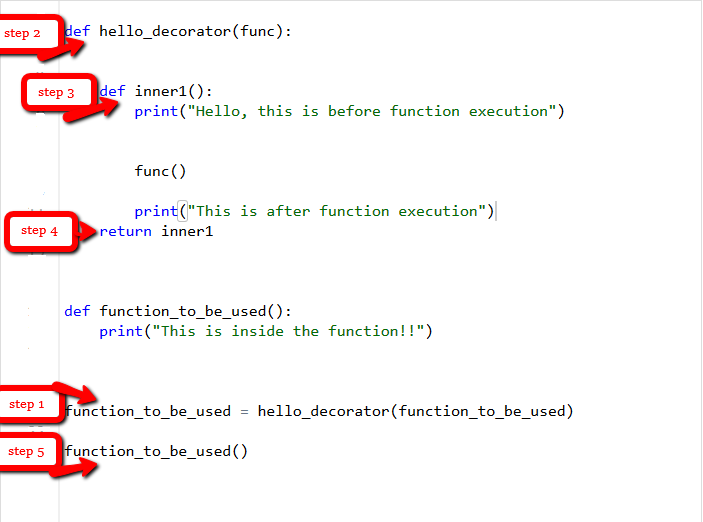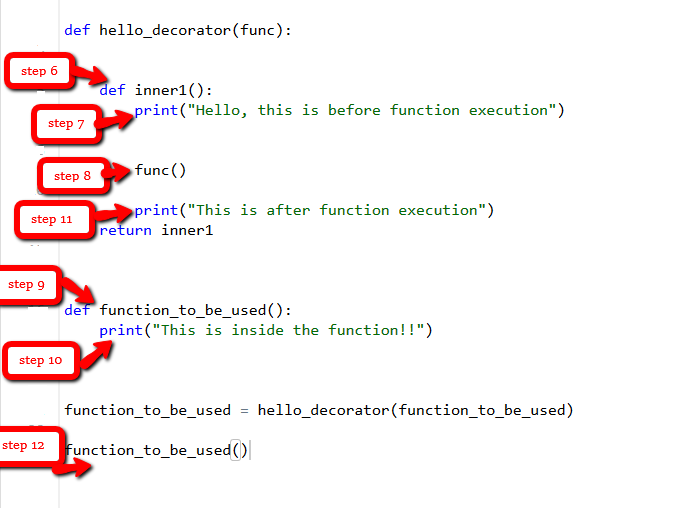# Decorators in Python

In Python, functions are the first class objects, which means that –

• Functions are objects; they can be referenced to, passed to a variable and returned from other functions as well.
• Functions can be defined inside another function and can also be passed as argument to another function.

Decorators are very powerful and useful tool in Python since it allows programmers to modify the behavior of function or class. Decorators allow us to wrap another function in order to extend the behavior of wrapped function, without permanently modifying it.

In Decorators, functions are taken as the argument into another function and then called inside the wrapper function.

Syntax for Decorator:

 `@gfg_decorator` `def` `hello_decorator(): ` `    ``print``(``"Gfg"``) ` ` `  `'''Above code is equivalent to - ` ` `  `def hello_decorator(): ` `    ``print("Gfg") ` `     `  `hello_decorator = gfg_decorator(hello_decorator)'''`

In the above code, `gfg_decorator` is a callable function, will add some code on the top of some another callable function, `hello_decorator ` function and return the wrapper function.

Decorator can modify the behavior:

 `# defining a decorator ` `def` `hello_decorator(func): ` ` `  `    ``# inner1 is a Wrapper function in  ` `    ``# which the argument is called ` `     `  `    ``# inner function can access the outer local ` `    ``# functions like in this case "func" ` `    ``def` `inner1(): ` `        ``print``(``"Hello, this is before function execution"``) ` ` `  `        ``# calling the actual function now ` `        ``# inside the wrapper function. ` `        ``func() ` ` `  `        ``print``(``"This is after function execution"``) ` `         `  `    ``return` `inner1 ` ` `  ` `  `# defining a function, to be called inside wrapper ` `def` `function_to_be_used(): ` `    ``print``(``"This is inside the function !!"``) ` ` `  ` `  `# passing 'function_to_be_used' inside the ` `# decorator to control its behavior ` `function_to_be_used ``=` `hello_decorator(function_to_be_used) ` ` `  ` `  `# calling the function ` `function_to_be_used() `

Output:

```Hello, this is before function execution
This is inside the function !!
This is after function execution```

Let’s see the behavior of the above code how it runs step by step when the “function_to_be_used” is called.Let’s jump to another example where we can easily find out the execution time of a function using a decorator.

 `# importing libraries ` `import` `time ` `import` `math ` ` `  `# decorator to calculate duration ` `# taken by any function. ` `def` `calculate_time(func): ` `     `  `    ``# added arguments inside the inner1, ` `    ``# if function takes any arguments, ` `    ``# can be added like this. ` `    ``def` `inner1(``*``args, ``*``*``kwargs): ` ` `  `        ``# storing time before function execution ` `        ``begin ``=` `time.time() ` `         `  `        ``func(``*``args, ``*``*``kwargs) ` ` `  `        ``# storing time after function execution ` `        ``end ``=` `time.time() ` `        ``print``(``"Total time taken in : "``, func.__name__, end ``-` `begin) ` ` `  `    ``return` `inner1 ` ` `  ` `  ` `  `# this can be added to any function present, ` `# in this case to calculate a factorial ` `@calculate_time` `def` `factorial(num): ` ` `  `    ``# sleep 2 seconds because it takes very less time ` `    ``# so that you can see the actual difference ` `    ``time.sleep(``2``) ` `    ``print``(math.factorial(num)) ` ` `  `# calling the function. ` `factorial(``10``) `

Output:

```3628800
Total time taken in :  factorial 2.0061802864074707```

What if a function returns something

In all the above examples the functions didn’t return anything so there wasn’t any issue, but one may need the returned value.

 `def` `hello_decorator(func): ` `    ``def` `inner1(``*``args, ``*``*``kwargs): ` `         `  `        ``print``(``"before Execution"``) ` `         `  `        ``# getting the returned value ` `        ``returned_value ``=` `func(``*``args, ``*``*``kwargs) ` `        ``print``(``"after Execution"``) ` `         `  `        ``# returning the value to the original frame ` `        ``return` `returned_value ` `         `  `    ``return` `inner1 ` ` `  ` `  `# adding decorator to the function ` `@hello_decorator` `def` `sum_two_numbers(a, b): ` `    ``print``(``"Inside the function"``) ` `    ``return` `a ``+` `b ` ` `  `a, b ``=` `1``, ``2` ` `  `# getting the value through return of the function ` `print``(``"Sum ="``, sum_two_numbers(a, b)) `

Output:

```before Execution
Inside the function
after Execution
Sum = 3```

My Personal Notes arrow_drop_upCheck out this Author's contributed articles.

If you like GeeksforGeeks and would like to contribute, you can also write an article using contribute.geeksforgeeks.org or mail your article to contribute@geeksforgeeks.org. See your article appearing on the GeeksforGeeks main page and help other Geeks.

Please Improve this article if you find anything incorrect by clicking on the "Improve Article" button below.

Article Tags :

18

Please write to us at contribute@geeksforgeeks.org to report any issue with the above content.